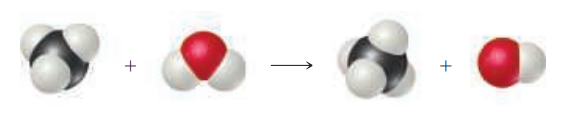×
Get Full Access to Chemistry: The Central Science - 14 Edition - Chapter 22 - Problem 22.2
Get Full Access to Chemistry: The Central Science - 14 Edition - Chapter 22 - Problem 22.2

×ISBN: 9780134414232 1274

## Solution for problem 22.2 Chapter 22

Chemistry: The Central Science | 14th Edition

• Textbook Solutions
• 2901 Step-by-step solutions solved by professors and subject experts
• Get 24/7 help from StudySoup virtual teaching assistantsChemistry: The Central Science | 14th Edition

4 5 1 344 Reviews
12
1
Problem 22.2

(a) Identify the type of chemical reaction represented by the following diagram.

(b) Place appropriate charges on the species on both sides of the equation.

(c) Write the chemical equation for the reaction. [Section 22.1]Step-by-Step Solution:
Step 1 of 3

Chapter 6 -Atoms: Electrons The world is made up different chemical species, and those species in return are made up of atoms. So, in return, atoms make up everything, but what makes up an atom Atoms are made up of 3 subatomic particles, the proton, carries a positive charge of +1, and the neutron make up the nucleus. Electrons carry a negative charge of -1 and they orbit the nucleus in different energy levels and shells and subshells. The type of chemical species built depends on both the physical and the chemical properties of the atom. Consequently, these properties are dependent on the energy levels and the arrangement of the electrons in the atom. Now in a common demonstration in class, you make a solution of ethanol and some metal ion, and set it on fire. Then you observe the flame produced, and you could clearly see different colours. This is known as the flame test. Why do different metal ions have Lithium: Deep red Strontium: red-orange Calcium: Orange Sodium: Bright yellow different flame colours Different metals emit different wavelengths and Barium: Green-yellow Copper: Green-blue frequencies of lights. This happens as energy is absorbed by the electron of Lead: light blue Potassium: violet the atom, exciting the electrons and raising them to a higher energy level that is then unstable. The electron cannot remain in that level as it is unstable, so the electron drops back to its ground state, the difference in energy is then rel

Step 2 of 3

Step 3 of 3

##### ISBN: 9780134414232

This full solution covers the following key subjects: . This expansive textbook survival guide covers 29 chapters, and 2820 solutions. This textbook survival guide was created for the textbook: Chemistry: The Central Science, edition: 14. The answer to “?(a) Identify the type of chemical reaction represented by the following diagram. (b) Place appropriate charges on the species on both sides of the equation. (c) Write the chemical equation for the reaction. [Section 22.1]” is broken down into a number of easy to follow steps, and 35 words. Chemistry: The Central Science was written by and is associated to the ISBN: 9780134414232. Since the solution to 22.2 from 22 chapter was answered, more than 205 students have viewed the full step-by-step answer. The full step-by-step solution to problem: 22.2 from chapter: 22 was answered by , our top Chemistry solution expert on 10/03/18, 06:29PM.

## Discover and learn what students are asking

Calculus: Early Transcendental Functions : Functions of Several Variables
?In Exercises 15 - 22, find all first partial derivatives. $$f(x, y)=y^{3} e^{4 x}$$

Calculus: Early Transcendental Functions : Change of Variables: Polar Coordinates
?Describing a Region In Exercises 5-8, use polar coordinates to describe the region shown.

Statistics: Informed Decisions Using Data : Goodness-of-Fit Test
?Explain why chi-square goodness-of-fit tests are always right tailed.

Unlock Textbook Solution